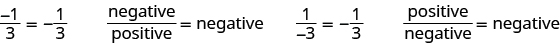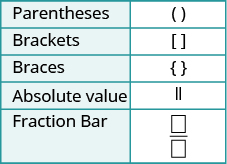## Simplifying an Expression With a Fraction Bar

### Learning Outcomes

• Identify negative fractions that are equivalent given that their negative sign is in a different location
• Simplify expressions that contain fraction bars using the order of operations

Where does the negative sign go in a fraction? Usually, the negative sign is placed in front of the fraction, but you will sometimes see a fraction with a negative numerator or denominator. Remember that fractions represent division. The fraction $\Large-\frac{1}{3}$ could be the result of dividing $\Large\frac{-1}{3}$, a negative by a positive, or of dividing $\Large\frac{1}{-3}$, a positive by a negative. When the numerator and denominator have different signs, the quotient is negative.If both the numerator and denominator are negative, then the fraction itself is positive because we are dividing a negative by a negative.

$\Large\frac{-1}{-3}\normalsize=\Large\frac{1}{3}\quad\quad\normalsize\Large\frac{\text{negative}}{\text{negative}}=\text{positive}$

For any positive numbers $a\text{ and }b$,

$\Large\frac{-a}{b}\normalsize=\Large\frac{a}{-b}\normalsize=\Large-\frac{a}{b}$

### Example

Which of the following fractions are equivalent to $\Large\frac{7}{-8}$?

$\Large\frac{-7}{-8},\frac{-7}{8},\frac{7}{8},-\frac{7}{8}$

Solution:
The quotient of a positive and a negative is a negative, so $\Large\frac{7}{-8}$ is negative. Of the fractions listed, $\Large\frac{-7}{8},-\frac{7}{8}$ are also negative.

## Simplifying an Expression With a Fraction Bar

Fraction bars act as grouping symbols. The expressions above and below the fraction bar should be treated as if they were in parentheses. For example, $\Large\frac{4+8}{5 - 3}$ means $\left(4+8\right)\div\left(5 - 3\right)$. The order of operations tells us to simplify the numerator and the denominator first—as if there were parentheses—before we divide.

We’ll add fraction bars to our set of grouping symbols from Use the Language of Algebra to have a more complete set here.

### Grouping Symbols### Simplify an expression with a fraction bar

1. Simplify the numerator.
2. Simplify the denominator.
3. Simplify the fraction.

### Example

Simplify: $\Large\frac{4+8}{5 - 3}$

### Try It

the following video provides another example of how to simplify various expressions that contain a fraction bar.

### Example

Simplify: $\Large\frac{4 - 2\left(3\right)}{{2}^{2}+2}$

### Example

Simplify: $\Large\frac{{\left(8 - 4\right)}^{2}}{{8}^{2}-{4}^{2}}$

### Example

Simplify: $\Large\frac{4\left(-3\right)+6\left(-2\right)}{-3\left(2\right)-2}$

### Try It

Watch this video to see another example of how to simplify an expression with a fraction bar that contains several different operations.